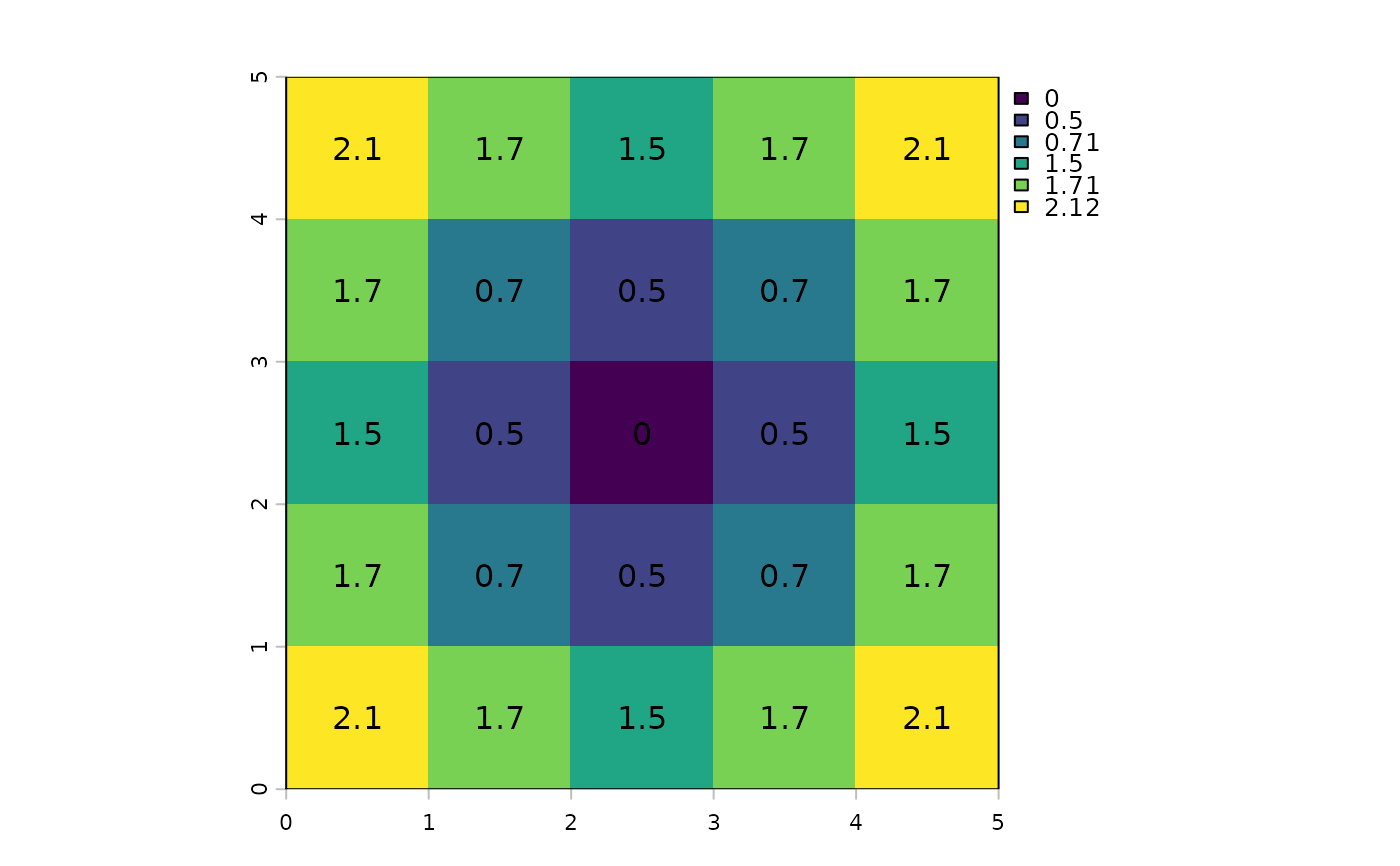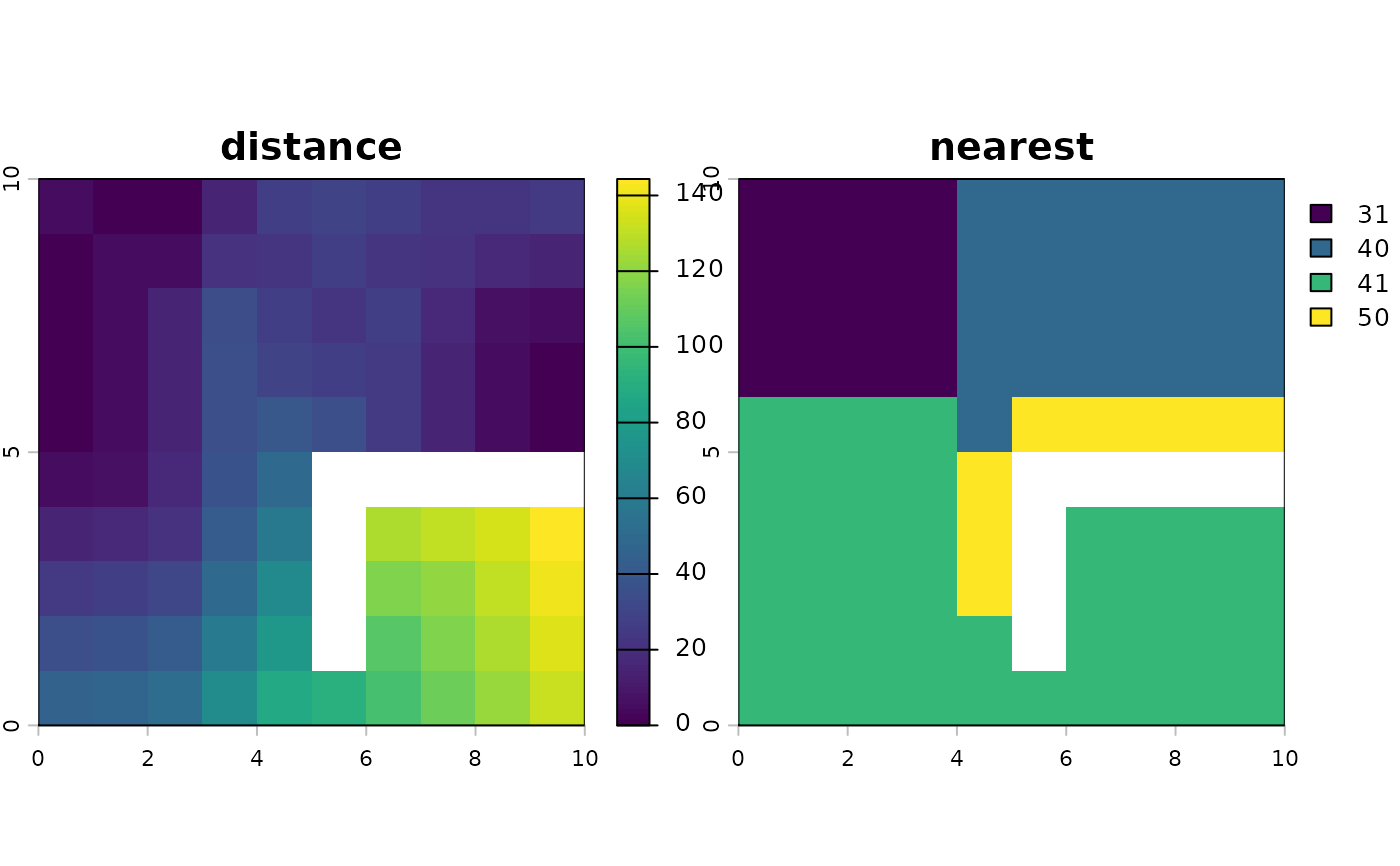Use a friction (cost) surface to compute the cost-distance from any cell to the border of one or more target cells.

Distances are computed by summing local distances between cells, which are connected with their neighbors in 8 directions, and assuming that the path has to go through the centers of one of the neighboring raster cells.

Distances are multiplied with the friction, thus to get the cost-distance, the friction surface must express the cost per unit distance (speed) of travel.

# S4 method for SpatRaster
costDist(x, target=0, scale=1, maxiter=50, filename="", ...)

## Arguments

x

SpatRaster

target

numeric. value of the target cells (where to compute cost-distance to)

scale

numeric. Scale factor. The cost distance is divided by this number

maxiter

numeric. The maximum number of iterations. Increase this number if you get the warning that costDistance did not converge

filename

character. output filename (optional)

...

additional arguments as for writeRaster

gridDist, distance

SpatRaster

## Examples

r <- rast(ncols=5, nrows=5, crs="+proj=utm +zone=1 +datum=WGS84",
xmin=0, xmax=5, ymin=0, ymax=5, vals=1)
r <- 0
d <- costDist(r)
plot(d)
text(d, digits=1)r <- rast(ncols=10, nrows=10,  xmin=0, xmax=10, ymin=0, ymax=10,
vals=10, crs="+proj=utm +zone=1 +datum=WGS84")
r[5, 1] <- -10
r[2:3, 1] <- r[1, 2:4] <- r[2, 5] <- 0
r[3, 6] <- r[2, 7] <- r[1, 8:9] <- 0
r[6, 6:10] <- NA
r[6:9, 6] <- NA

d <- costDist(r, -10)
plot(d)
text(d, digits=1, cex=.8)